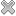# Courses / Module

Toggle Print

##DATA ANALYSIS (R)

Module code: ST821S
Credits: 5
Semester: 1
Department: MATHEMATICS AND STATISTICS
International:Overview

The course is case-study based and involves lots of practical work using R. Exploratory data analysis: summaries and graphics. Mosaic plots. Fisher�s exact test. Calculating probabilities, quantiles. Assessing Normality, Normal probability plots. Transformations. Simulation of CLT. Analysing data using one and two sample t-tests and confidence intervals. Correlation, simple linear regression inference and anova.

Lectures and tutorials as for ST201.

For Registered Research students. Permission is required from the Head of the Mathematics & Statistics Department.Learning OutcomesTeaching & Learning methodsAssessmentRepeat optionsPre-RequisitesTimetable